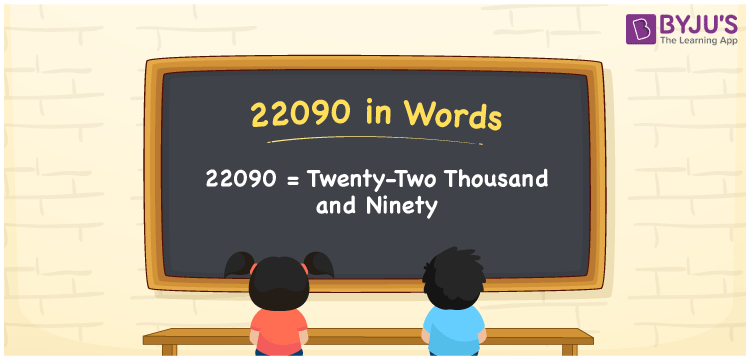# 22090 in Words

22090 in words is Twenty-two thousand and ninety. The number 22090 is a natural number and it exists between 22089 and 22091. It is also a cardinal number since it is specific to quantity. For converting the number 22090 into words, we use the place value system. Now, let us learn the spelling of 22090 in English words in this article.

 22090 in Words: Twenty-two Thousand and Ninety. Twenty-two Thousand and Ninety in Numerical Form: 22090.

## 22090 in English Words## How to Write 22090 in Words?

The place value table for 22090 is given below:

 Ten-thousands Thousands Hundreds Tens Ones 2 2 0 9 0

The expanded form of 22090 is as follows:

= 2 × Ten thousand + 2 × Thousand + 0 × Hundred + 9 × Ten + 0 × One

= 2 × 10000 + 2 × 1000 + 0 × 100 + 9 × 10 + 0 × 1

= 20000 + 2000 + 90

= 22090

= Twenty-two thousand and ninety

Hence, 22090 in words is twenty-two thousand and ninety.

22090 in words – Twenty-two thousand and ninety

Is 22090 an odd number? – No

Is 22090 an even number? – Yes

Is 22090 a perfect square number? – No

Is 22090 a perfect cube number? – No

Is 22090 a prime number? – No

Is 22090 a composite number? – Yes

## Frequently Asked Questions on 22090 in Words

Q1

### How to spell 22090?

22090 in words is twenty-two thousand and ninety.

Q2

### Simplify 22000 + 90, and express it in words.

Simplifying 22000 + 90, we get 22090. Hence, 22090 in words is twenty-two thousand and ninety.

Q3

### How to write twenty-two thousand and ninety in numbers?

Twenty-two thousand and ninety in numbers is 22090.